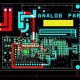# How to Calculate Impedance of Pair Trace in High Speed PCB?

In this tutorial we are going to learn about How to Calculate Impedance on a Given Pair of Trace in High Speed PCB?

In High Speed PCB , The transmission line of a characteristic impedance is dependent on the relationship of the conductor width, conductor thickness, dielectric thickness between conductor & GND-PWR reference planes & the dielectric constant of the dielectric medium. The actual impedance can be tested via a small prototype build. It is generally necessary when tight impedance tolerances are required or in the case of small line widths & dielectric thicknesses, which are more sensitive to variations. The tolerance swing due to etching variations will be more significant for a 0.127 mm (0.005 inch) line width than for a 0.254 mm(0.010 inch) line, for example. The recommended impedance tolerance is 10 %. A lesser tolerance is often achievable, especially with fully embedded Microstrip & Stripline structures. The Microstrip line is important transmission line structure for high speed digital circuits. Always in PCB design have surface Microstrip location on the external layer is subject to potentially greater impedance variables. This is due to the additional copper electroplating it receives, resulting in increased line thickness & line width tolerances. For critical applications, the Microstrip line may have to be   embedded in dielectric material. The impedance can be calculated from the Surface Microstrip formula. Then for each 0.001 inch below the surface, subtract 1% of the impedance calculated and it is de rating factor provides good results for  embedding up to approximately 0.381 mm. A thicker embedding has little additional effect. The strip line is embedded in dielectric material & is sandwiched between 2 reference planes. This configuration significantly reduces cross talk effect. This structure is most suitable for improving impedance tolerances.

The Impedance is inversely proportional to trace width Impedance is inversely proportional to trace thickness Impedance is proportional to laminate height Impedance is inversely proportional to the square root of laminate Dielectric Constant(Er)

a) For two traces next to each other the following formula applies [10, 11].

ZO = 120 1n(p.h/(b+c))

v ? r

Where h= distance between traces

B= width of the trace

C= Thickness of the trace; typical 17mm

b) for two traces on top of each other:

ZO = 120 p (h/h+b))

v ? r

where h=1.5mm(typical thickness of epoxy)

c) When the trace is above a goundplane the following formula applies:

ZO = 87 ln(6.h/(0.8.b+c))

v (?r+v 2)

d) and in case of a trace between two (ground) planes the formula yields:

ZO = 60 ln(4k/(0.67. p.b(0.8+b/c)))

v ? r

where K= distance in-between the planes.

Typically the permittivity for epoxy material is ?r =4.7## About EEE

We have designing Experience for the last 40 years.